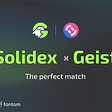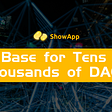# 1. Brief Introduction

1. Computing tasks in this testnet are generated virtually by the system. A fixed 5 computing tasks will be created for each Round (600 blocks). The amount of mining rewards is consistent with the white paper. (50% of the rewards for each block mined will be obtained by TEEs that perform privacy computing tasks, 30% of the rewards will be obtained by all online TEEs, and 20% will flow into the Phala treasury.)
2. In the Vendetta testnet, the system automatically stakes the minimum stake amount for each CPU, which is 0 tPHA per core.
3. Miners can voluntarily use tPHA as an additional stake for their own CPU, and anyone can also use their own tPHA as a stake for other miners, but the settlement does not support automatic distribution to the nominator at this time.
4. Within 1 Round, 1 TEE can be assigned only 1 computation task.
5. The algorithm and parameters of computing tasks distribution in the testnet are experimental and will be upgraded after the mainnet goes online; the settlement method for rewards may also change.

# 2.Privacy Computing Tasks Dispatch Algorithm

## 2.1 Core logic

1. Like any dispatch algorithm, we need to assign the suitable TEEs to perform the computation based on the characteristics of the privacy computing tasks;
2. The Phala Network system will score each TEE device according to its computing characteristics;
3. Based on the scores of all online TEEs, the assignment result is calculated by weighted random sampling formula;
4. After the assigned TEE(s) complete the privacy computation, the system will automatically settle and issue rewards. To ensure system security, rewards will be frozen for a period of time. (Rewards issued by the Vendetta testnet are Fire II, which are only used for the statistics of the settlement prize pool and cannot be transferred).

## 2.2 Metrics for TEE Calculation and Dispatch

The probability of a TEE being assigned a computing task depends on two characteristics of the CPU: computing power and security.

1. The computing power is represented by the CPU score. In the future, these will be set per CPU type through on-chain voting (no-fork upgrade method);

2. Security is based on the CPU core’s stake amount.

Increased computing power and security do not linearly increase the probability of a TEE worker obtaining computing tasks. (See Section 3 for more information.)

## 2.3 TEE Worker Gets a Weight for the Probability of Dispatch

Using the metrics, we can calculate a weighted score for each TEE.

The formula for calculating the weight is:

1. Score= TEE computing performance score

2. Stake = TEE stake amount - basic stake amount (In Vendetta testnet the basic stake amount is 0)

## 2.4 Weighted Random Sampling Without Replacement

We use weighted random sampling without replacement (meaning each TEE can only be sampled once), and randomly sample n TEEs from all m online TEEs to perform computation tasks.

Weighting means that the weighted score w of each TEE is used as the basic value in the sampling, that is, the higher the w, the higher the probability of winning.

An example of weighted random sampling without replacement:

Suppose there are three machines A, B, and C.

Machine A’s score is 3, machine B’s score is 2, and Machine C’s score is 1.

Now, drawing one from the 3 machines, the probability of A being drawn is:

If two sets are drawn, the probability of A being drawn is:

Depending on participation in 1605 Race V1, there may be 5 tasks assigned to thousands or tens of thousands of TEEs in the real environment, but the logic is consistent with the above example.

In order to allow TEE miners to predict their own probability of winning and to simulate the relationship between the amount of stake tPHA and the probability of winning, we will provide a privacy computation probability calculator on the Dashboard (the calculator tutorial will be released in days), and miners can fill in their own parameters. The performance and expected stake amount simulate the probability of order placement.

# 3. Data Simulation of Dispatching Algorithm

## 3.1 The Correlation Between Extra Stake and the Probability of Selection

Assuming that the TEE scores are consistent, what is the impact of different stake amounts on the selection probability?

The algorithm is clear, but based on the algorithm alone, it is impossible to calculate the probability of a single mining machine being drawn, because the probability of drawing is related to the number of mining machines currently online and their task points. We simulated the increase in the probability of winning by the additional stake amount.

Assume that other TEEs are 5000 units of 420 — score machines, and they all have an additional 1000 tPHA stake. Rorschach also has a similar machine, so there are 5001. If 5 machines are selected from the 5001 to perform computing tasks, then as Rorschach adds additional stakes, the probability of being drawn increases as follows:

As can be seen from the graph:

1. The probability of drawn as one of the 5 is very low, about 0.05%;

2. As the amount of additional stake increases, the probability increases rapidly at first, and then slowly;

3. But even if the stake amount increases to 20,000, the winning rate only increases to 0.17%.

## 3.2 The Correlation Between Machine Performance and Selection Probability

In the above picture, the light blue, red, yellow, and green lines are machines with scores of 400, 300, 200, and 100 points respectively. It can be seen that the machine performance maintains a constant absolute advantage in the probability of winning.

## 3.3 How should multiple miners allocate additional stakes?

Assume that Rorschach has two identical mining machines and has a fixed amount to dedicate as additional stake. How the amount is apportioned does not make a big difference, but splitting the amount evenly between them will provide the highest odds.

The simulated situation in the figure below is that Rorschach has two TEEs with 420-point computing scores, and both have staked 5000 tPHA. The other 5000 TEEs in the system have 1000 tPHA stakes, and they are all 420 points.

If Rorschach has 20,000 additional tPHA to use for staking,

• The leftmost side of the X-axis in the figure below represents assigning all 20,000 tPHA to one TEE, and the probability of getting a task is 0.28%;
• The far-right represents assigning all 20000 additional tPHA to the other TEE, which is also 0.28% likely to get a task;
• The middle section represents the equal distribution of stake to both TEEs. The probability of getting a task is now 0.32%.

we can see that the odds are slightly higher than when assigning all the tPHA to just one.

Phala Network is a light, powerful, and secure confidential smart contract platform which runs contracts in trusted hardware enclaves inside processors, creating a novel privacy computing cloud with private computing and services for both users and companies.

Phala Network will be a parachain on Polkadot and a solution to our next-generation privacy needs. Member of the Confidential Computing Consortium (CCC). Recipient of 2 Web3 Foundation Grants.

--

--

--

## More from Phala Network

Phala Network is a privacy-preserving cloud computing service, based on Substrate, and will run as a parachain in the Polkadot ecosystem to offer computing power comparable to existing cloud services but which protects the privacy of managed programs.

## The Future of Transfer Pricing: Blockchain## Khala Network Has Successfully Registered the 4th Kusama Parachain ID｜Phala Weekly Vol.34## The Barrier to DeFi Growth — Seed Phrase?## Minxie is The Web3 Answer for Creator Economy## Geist Finance partnership with Solidex## On-ledger, Recurring Developer Revenue. Incentives to Buidl.## Show Platform, A Base for Tens of Thousands of DAOs## Phala Network

Infrastructure of Web3.0 privacy protection

## peaq joins the Mobility Open Blockchain Initiative (MOBI)## Standard Protocol Partner Showcase — Ankr## How Vulture will stand out## Three Arrows Capital Defaults on \$670 Million Loan, Heightening Contagion Risks